Next: MATRIX FILTERS, SPECTRA, AND Up: Matrices and multichannel time Previous: REVIEW OF MATRICES

# SYLVESTER'S MATRIX THEOREM

Sylvester's theorem provides a rapid way to calculate functions of a matrix. Some simple functions of a matrix of frequent occurrence are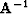and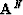(for N large). Two more matrix functions which are very important in wave propagation areand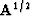.Before going into the somewhat abstract proof of Sylvester's theorem, we will take up a numerical example. Consider the matrix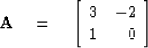(26)
It will be necessary to have the column eigenvectors and the eigenvalues of this matrix; they are given by(27) (28)
Since the matrix A is not symmetric, it has row eigenvectors which differ from the column vectors. These are(29) (30)
We may abbreviate equations (27) through (30) by(31)
The reader will observe that r or c could be multiplied by an arbitrary scale factor and (31) would still be valid. The eigenvectors are said to be normalized if scale factors have been chosen so that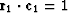and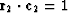.It will be observed thatand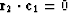,a general result to be established in the exercises.

Let us consider the behavior of the matrix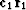.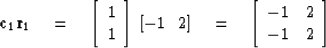Any power of this matrix is the matrix itself, for example its square.This property is called idempotence (Latin for self-power). It arises because.The same thing is of course true of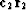. Now notice that the matrixis perpendicular'' to the matrix, that is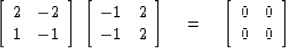since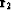andare perpendicular.

Sylvester's theorem says that any function f of the matrix A may be written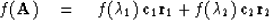The simplest example is(32)
Another example is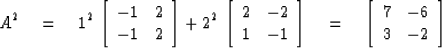The inverse isThe identity matrix may be expanded in terms of the eigenvectors of the matrix A.Before illustrating some more complicated functions let us see what it takes to prove Sylvester's theorem. We will need one basic result which is in all the books on matrix theory, namely, that most matrices (see exercises) can be diagonalized. In terms of our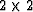example this takes the form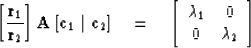(33)
where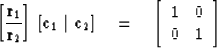(34)
Since a matrix commutes with its inverse, (34) implies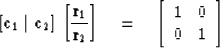(35)
Postmultiply (33) by the row matrix and premultiply by the column matrix. Using (35), we get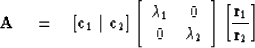(36)
Equation (36) is (32) in disguise, as we can see by writing (36) as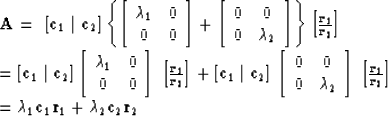Now to getwe have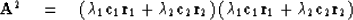Using the orthonormality ofandthis reduces toIt is clear how (36) can be used to prove Sylvester's theorem for any polynomial function of A. Clearly, there is nothing peculiar aboutmatrices either. This works for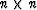.Likewise, one may consider infinite series functions in A. Since almost any function can be made up of infinite series, we can consider also transcendental functions like sine, cosine, exponential.

Exponentials arise naturally as the solutions to differential equations. Consider the matrix differential equation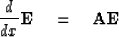(37)
One may readily verify the power series solution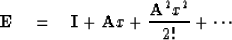This is the power series definition of an exponential function. If the matrix A is one of that vast majority which can be diagonalized, then the exponential can be more simply expressed by Sylvester's theorem. For the numerical example we have been considering, we have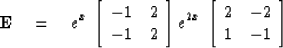The exponential matrix is a solution to the differential equation (37) without regard to boundaries. It frequently happens that physics gives one a differential equation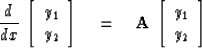(38)
Subject to two boundary conditions on either of y1 or y2 or a combination. One may verify that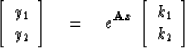is the solution to (38) for arbitrary constants k1 and k2. Boundary conditions are then used to determine the numerical values of k1 and k2. Note that k1 and k2 are just y2 (x = 0) and y2 (x = 0).

An interesting situation arises with the square root of a matrix. Amatrix like A will have four square roots because there are four possible combinations for choice of plus or minus signs on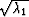and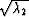.In general, anmatrix has 2n square roots. An important application arises in a later chapter, where we will deal with the differential operator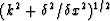.The square root of an operator is explained in very few books and few people even know what it means. The best way to visualize the square root of this differential operator is to relate it to the square root of the matrix M whereThe right-hand matrix is a second difference approximation to a second partial derivative. Let us defineClearly we wish to consider M generalized to a very large size so that the end effects may be minimized. In concept, we can make M as large as we like and for any size we can getsquare roots. In practice there will be only two square roots of interest, one with the plus roots of all the eigenvalues and the other with all the minus roots. How can we find these principal value'' square roots? An important case of interest is where we can use the binomial theorem so that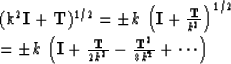The result is justified by merely squaring the assumed square root. Alternatively, it may be justified by means of Sylvester's theorem. It should be noted that on squaring the assumed square root one utilizes the fact that I and T commute. We are led to the idea that the square root of the differential operator may be interpreted as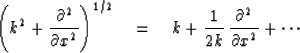provided that k is not a function of x. If k is a function of x, the square root of the differential operator still has meaning but is not so simply computed with the binomial theorem.

## EXERCISES:

1. Premultiply (31)b by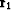and postmultiply (31)c by,then subtract. Is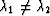a necessary condition forandto be perpendicular? Is it a sufficient condition?
2. Show the Cayley-Hamilton theorem, that is, if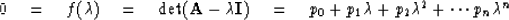then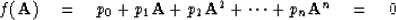3. Verify that, for a generalmatrix A, for which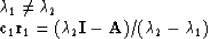where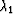and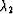are eigenvalues of A. What is the general form for?
4. For a symmetric matrix it can be shown that there is always a complete set of eigenvectors. A problem sometimes arises with nonsymmetric matrices. Study the matrix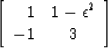as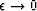to see why one eigenvector is lost. This is called a defective matrix. (This example is from T. R. Madden.)
5. A wide variety of wave-propagation problems in a stratified medium reduce to the equation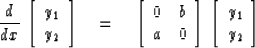What is the x dependence of the solution when ab is positive? When ab is negative? Assume a and b are independent of x. Use Sylvester's theorem. What would it take to get a defective matrix? What are the solutions in the case of a defective matrix?
6. Consider a matrix of the formwhere v is a column vector and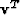is its transpose. Findin terms of a power series in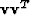.[Note that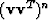collapses totimes a scaling factor, so the power series reduces considerably.]
7. The following cross-product'' matrix often arises in electrodynamics. Let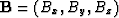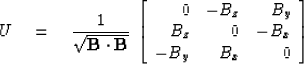(a)
Write out elements of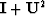.
(b)
Show thator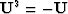.

(c)
Let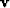be an arbitrary vector. In what geometrical directions do Uv,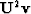, and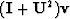point?

(d)
What are the eigenvalues of U? [Hint:  Use part (b).]

(e)
Why cannot U be canceled from?

(f)
Verify that the idempotent matrices of U areNext: MATRIX FILTERS, SPECTRA, AND Up: Matrices and multichannel time Previous: REVIEW OF MATRICES
Stanford Exploration Project
10/30/1997